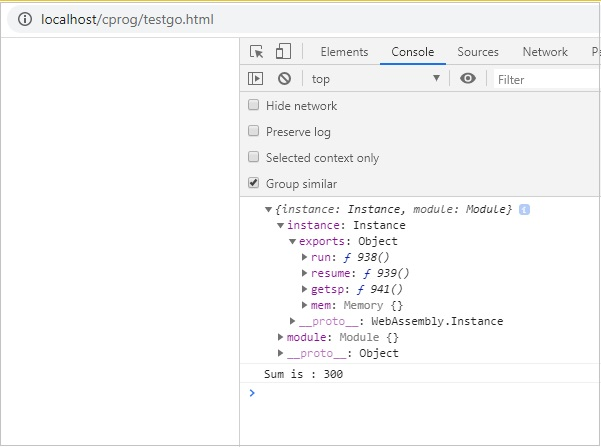# WebAssembly - Working with Go

Once done, write a simple program that adds two numbers in go.

testnum.go

```package main
import "fmt"
func main() {
var a int = 100
var b int = 200
var ret int
ret = sum(a, b)
fmt.Printf( "Sum is : %d\n", ret )
}

/* function returning the max between two numbers */
func sum(num1, num2 int) int {
return num1+num2
}
```

To compile above code to wasm, first set the environment variables in Go.

You will have to run following command −

```Set GOOS=js
GOARCH=wasm
```

Once done, execute the below command −

```go build -o testnum.wasm testnum.go
```

You should get testnum.wasm file once the command is executed.

Let us now test the code in the browser. To do that, we need to get the wasm_exec.js, that is installed with go.

The file wasm_exec.js will be available inside misc/wasm/ folder in go.

## Example

Here, is the code for testgo.html that makes use of wasm_exec.js and testnum.wasm.

```<html>
<meta charset="utf-8"/>
<script src="wasm_exec.js"></script>
<body>
<script type="text/javascript">
const importObj = {
module: {}
};
const go = new Go();
async function fetchAndInstantiate() {
const response = await fetch("testnum.wasm");
const buffer = await response.arrayBuffer();
const obj = await WebAssembly.instantiate(buffer, go.importObject);
console.log(obj);
go.run(obj.instance);
}
fetchAndInstantiate();
</script>
</body>
</html>
```

## Output

The output is as follows −